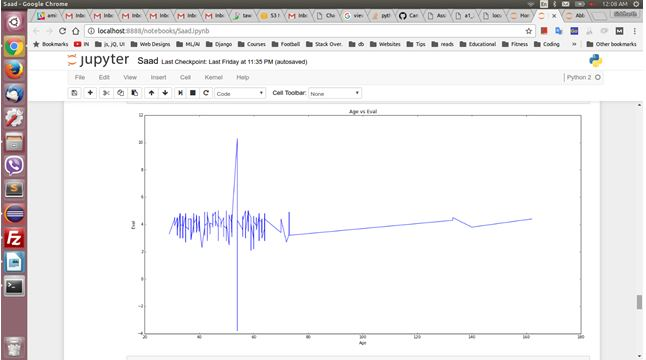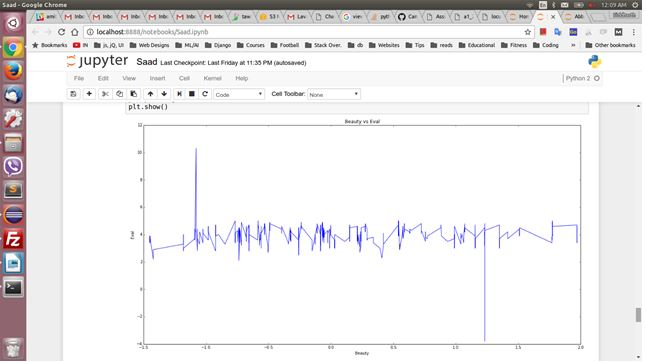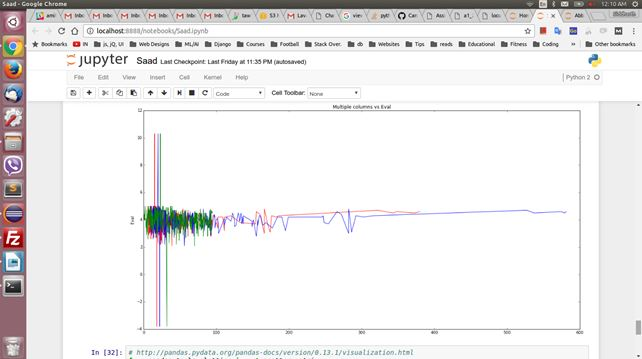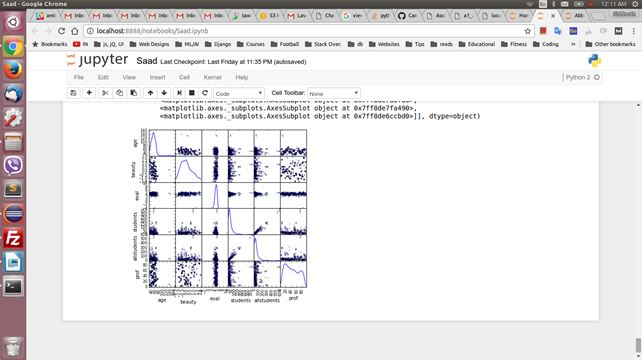COSC2670 Practical Data Science Proof Reading ServicesData Preparation

In order to correctly analyse data, we need to make sure that the data provided doesn’t have any errors involved. So, we need to check for the inconsistency in data and resolve them using appropriate techniques. Issues can be empty values, whitespaces in data, case sensitive data etc.

So, we need to identify and clean the data to make accurate analysis.

1.First task was to read the data from file. The csv file was having headers at the top followed by the actual data. Hence, we used the pandas read_csv function to read the data and ignoring the headers.

2. On checking the datatypes for all columns, we found the following:

minority        object

age            float64

gender          object

credits         object

beauty         float64

eval           float64

division        object

native          object

tenure          object

students         int64

allstudents      int64

prof             int64

dtype: object

Filling Incorrect/Missing Data

1.For columns involving strings, we found some typos in the provided data e.g. 'yesd' for 'yes' in minority column. Hence, we used pandas replace() function to replace them with correct values.

2.For numerical columns, we filled the NA values with the column 'mean'.

e.g.: data['eval'].fillna(data['eval'].mean(axis=0), inplace=True)

Data Exploration

Note: For 'Beauty' column data can be plotted by taking the approximation as the data is present upto 7 decimal places

Data for particular column

1.For 'Age' field we used a histogram to identify the age distribution as it is a numeric field.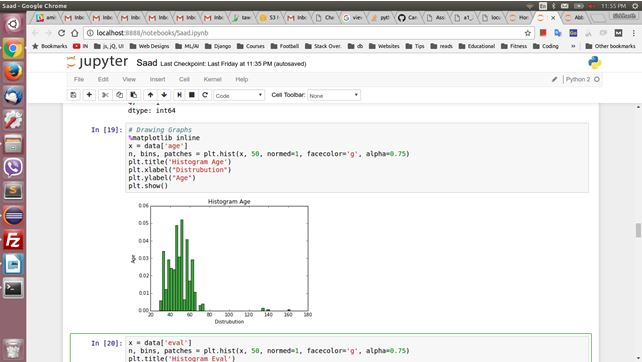2.For 'Eval' field we used a histogram to identify the eval distribution as it is a numeric field.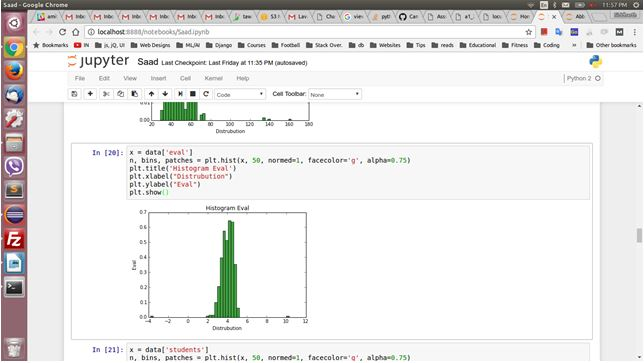3.For 'Students' field we used a histogram to identify the number of students distribution as it is a numeric field.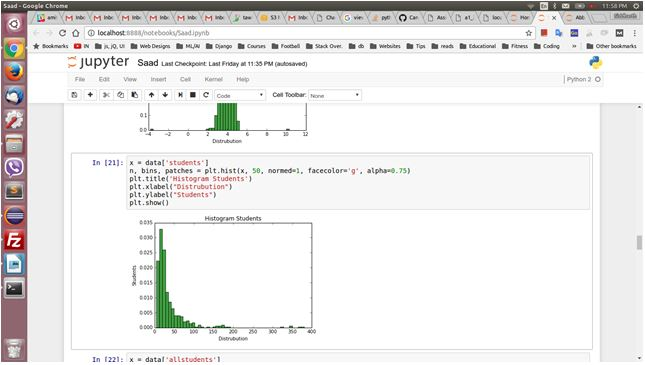4.For 'All Students' field we used a histogram to identify the number of all students distribution as it is a numeric field.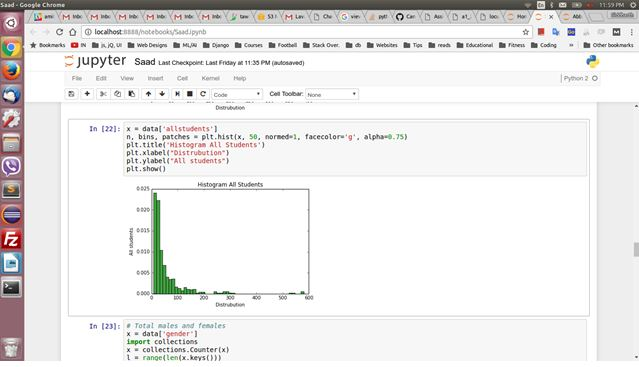5. For 'Gender' field we used a histogram to identify the gender as it is a choice field.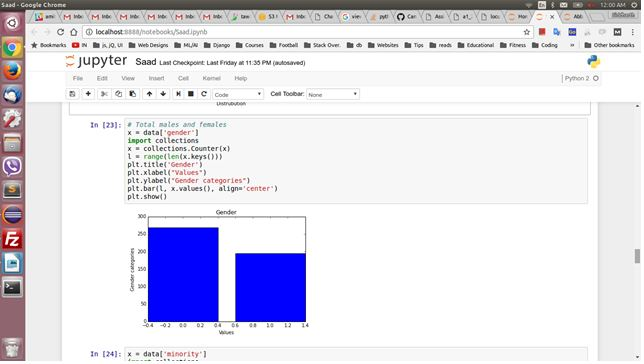6. For 'Minority' field we used a histogram to identify the minority as it is a choice field.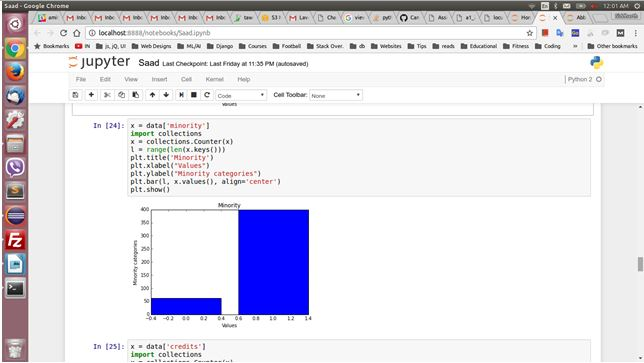7. For 'Credits' field we used a histogram to identify the credits as it is a choice field.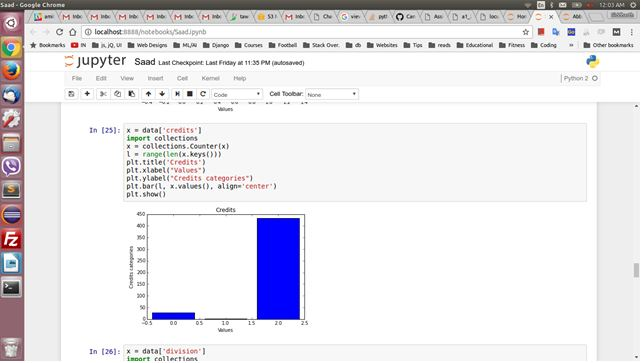8.For 'Division' field we used a histogram to identify the division as it is a choice field.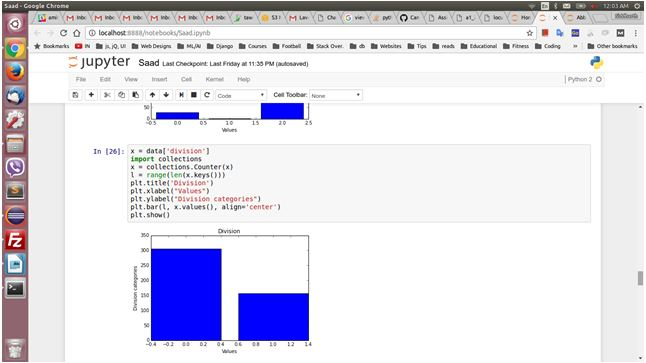9.For 'Native' field we used a histogram to identify the native as it is a choice field.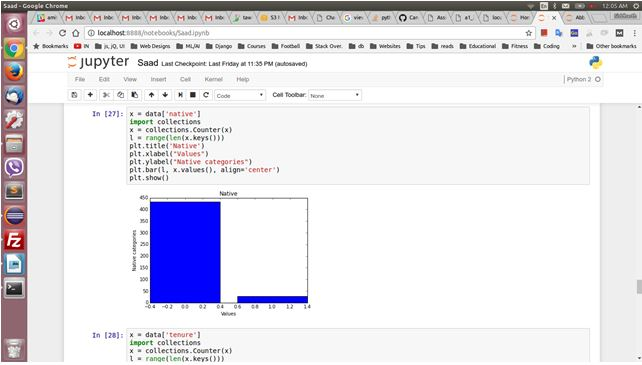10. For 'Tenure' field we used a histogram to identify the tenure as it is a choice field.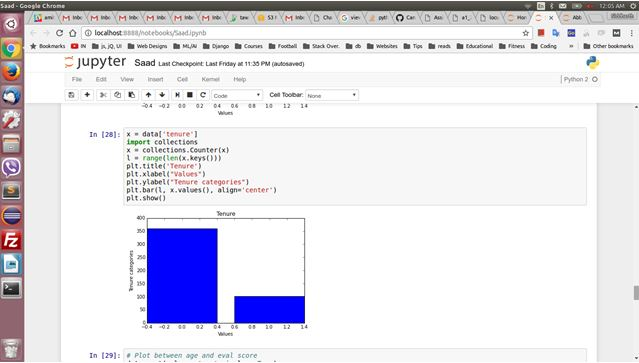Individual Plots

1. Age vs Eval

This plot will determine how age is influencing the eval score of a teacher. We will use a line plot to determine this.